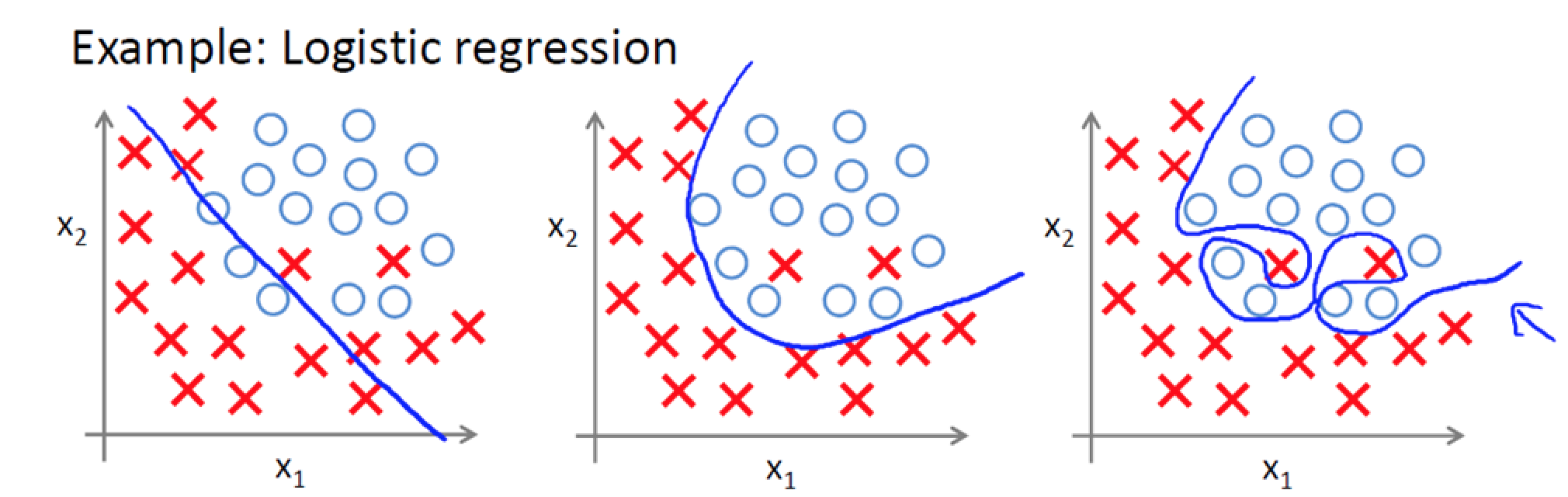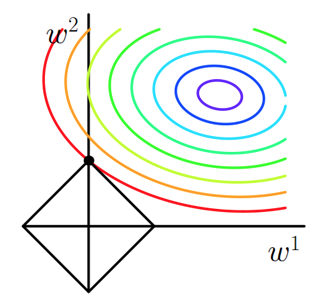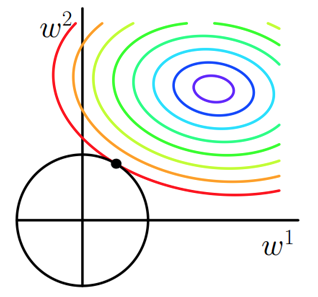# 深度神经网络之正则化

### 1.正则化

#### 1.1 为什么要正则化？• **欠拟合：**图1分类明显欠缺，有些男生被分为女生，有些女生被分为男生。
• **正拟合：**图2虽然有两个男生被分类为女生，但能够理解，毕竟我们人类自己也有分类错误的情况，比如通过化妆，女装等方法。
• 过拟合：图3虽然能够全部分类正确，但结果全部正确就一定好吗？不一定，我们能够看到分类曲线明显过于复杂，模型学习的时候学习了过多的参数项，但其中某些参数项是无用的特征，比如眼睛大小。当我们进行识别测试集数据时，就需要提供更多的特征，如果测试集包含海量的数据，模型的时间复杂度可想而知。

#### 1.2 什么是正则化？

f ( X ) = w 0 x 0 + w 1 x 1 + w 2 x 3 + . . . + w n x n f(X)=w_0x_0+w_1x_1+w_2x_3+...+w_nx_n

J 0 = ∣ ∣ f ( x ) − y ∣ ∣ 2 2 = ∣ ∣ X W − y ∣ ∣ 2 2 J_0=||f(x)-y||_2^2=||XW-y||^2_2

• **L0范数：**向量中非零元素的个数，记为 ∣ ∣ W ∣ ∣ 0 ||W||_0
• **L1范数：**绝对值之和，记为 ∣ ∣ W ∣ ∣ 1 ||W||_1
• **L2范数：**通常意义上的模，记为 ∣ ∣ W ∣ ∣ 2 ||W||_2

J = J 0 + L 1 = ∣ ∣ X W − y ∣ ∣ 2 2 + α ∣ ∣ W ∣ ∣ 1 J=J_0+L1=||XW-y||^2_2+\alpha||W||_1

J = J 0 + L 2 = ∣ ∣ X W − y ∣ ∣ 2 2 + α ∣ ∣ W ∣ ∣ 2 J=J_0+L2=||XW-y||^2_2+\alpha ||W||_2

#### 1.3 L1正则化和L2正则化

L1正则化可以产生稀疏值矩阵，即产生一个稀疏模型，可以用于特征选择和解决过拟合。那什么是稀疏值矩阵呢？稀疏矩阵是矩阵中很多元素为0，只有少数元素是非零值的矩阵，稀疏矩阵的好处就是能够帮助模型找到重要特征，而去掉无用特征或影响甚小的特征。

J = J 0 + L 1 = J 0 + α ∑ w ∣ w ∣ J=J_0+L_1=J_0+\alpha \sum_{w}|w|J = J 0 + L 2 = J 0 + λ 2 n ∑ w w 2 J=J_0+L_2=J_0+\frac{\lambda}{2n}\sum_{w}w^2

∂ J ∂ w = ∂ J 0 ∂ w + λ n w \frac{\partial J}{\partial w}=\frac{\partial J_0}{\partial w}+\frac{\lambda}{n}w

∂ J ∂ b = ∂ J 0 ∂ n \frac{\partial J}{\partial b}=\frac{\partial J_0}{\partial n}

w → w − α ∂ J 0 ∂ w − α λ n w w\rightarrow w-\alpha \frac{\partial J_0}{\partial w}-\frac{\alpha \lambda}{n}w

= ( 1 − α λ n ) w − α ∂ J 0 ∂ w =(1-\frac{\alpha \lambda}{n})w-\alpha\frac{\partial J_0}{\partial w}### 2.DNN之L1和L2正则化

J ( W , b ) = 1 2 m ∑ i = 1 m ∣ ∣ a L − y ∣ ∣ 2 2 J(W,b)=\frac{1}{2m}\sum _{i=1}^{m}||a^L-y||_2^2

J ( W , b ) = 1 2 m ∑ i = 1 m ∣ ∣ a L − y ∣ ∣ 2 2 + λ 2 m ∑ l = 2 L ∣ ∣ w ∣ ∣ 2 2 J(W,b)=\frac{1}{2m}\sum _{i=1}^{m}||a^L-y||_2^2+\frac{\lambda}{2m}\sum_{l=2}^{L}||w||_2^2

W l = W l − α ∑ i = 1 m δ i , l ( a x , l − 1 ) T W^l=W^l-\alpha \sum_{i=1}^{m} \delta^{i,l}(a^{x,l-1})^T

W l = W l − α ∑ i = 1 m δ i , l ( a x , l − 1 ) T − α m λ W l W^l=W^l-\alpha \sum_{i=1}^{m} \delta^{i,l}(a^{x,l-1})^T-\frac{\alpha }{m}\lambda W^l

### 3.DNN之Dropout正则化

Dropout指的是在用前向传播算法和反向传播算法训练模型时，随机的从全连接DNN网络中去掉一部分隐含层的神经元。比如我们完整的DNN模型如下所示### 4.DNN之集成学习正则化

DNN中我们采用的是若干个DNN的网络，首先对原始的训练样本进行有放回的随机采样，构建N组m个样本的数据集，然后分别用这N组数据集去训练我们的DNN。通过利用前向传播算法和反向传播算法得到N个DNN模型的W,b参数组合，然后对N个DNN模型的输出用加权平均法或者投票法决定最后的输出。最后，因为DNN模型比较复杂，通过Bagging后模型参数会增加N倍，从而导致训练模型需要花费较长的时间，因此一般N的取值不能太大，5-10个即可。

### 6.推广11-2309-161142
03-081万+
02-28270
05-111815
05-105392
09-089493
10-05418
06-30132
03-1411万+
09-304421
12-01229
04-081万+
08-092万+
06-14149
02-10948
09-141083
05-07770
©️2020 CSDN 皮肤主题: 技术黑板 设计师:CSDN官方博客